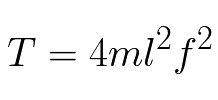# Belt Calculator

The vibration frequency of a plucked string is dependent upon the tension of that string. As the tension is increased, the vibration frequency also increases. Laboratory investigations show that power transmission belts react in a similar manner.

Data indicates that there is a direct relationship between belt tension and a belt’s natural frequency of vibration. This relationship holds true except for the very extreme high-tension zones (well above where any belt system can operate). Using load cells and accelerometers while applying Newtonian law, the linkage between strand tension and natural vibration frequency has been defined. It was found that unlike with a string, the mass of a belt does play a role in the results. The relationship between tension and frequency has been determined to be:String theory ignores flexural stiffness. A belt does have some stiffness so the calculated tension for a given frequency will be slightly higher than the actual tension. For belt spans greater than 0.25m, the above equation will provide results within 10% of the actual values. Beam analysis may give improved accuracy but the required inputs are generally too cumbersome for field application.

The Clavis Belt Tension Meter is a dual function tool. The sensor head uses either an invisible infrared beam or twin transducers to recover the
acoustic signal to detect vibration while the integral calculator determines the time base and performs the necessary calculations to support the results shown in the display window.

The Clavis Belt Tension Meter may be used with all power transmission belts regardless of type or construction.

## Calculate Belt Frequency

m - Belt mass per metre: kg/m
L - Belt span: metres
T - Belt tension:
f - Calculated Frequency:
Acceptable Frequency Range:

## Calculate Belt Tension

m - Belt mass per metre: kg/m
L - Belt span: metres
f - Belt Frequency:
T - Calculated Belt Tension:

## Calculate Belt Span

Span:
CD - Distance between the center of the pulleys:
D - Pulley 1 Diameter:
d - Pulley 2 Diameter: